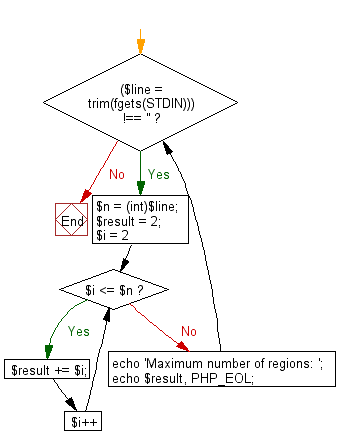﻿ PHP Exercise: Create maximum number of regions obtained by drawing n given straight lines - w3resource

# PHP Exercises: Create maximum number of regions obtained by drawing n given straight lines

## PHP: Exercise-67 with Solution

If you draw a straight line on a plane, the plane is divided into two regions. For example, if you pull two straight lines in parallel, you get three areas, and if you draw vertically one to the other you get 4 areas.
Write a PHP program to create maximum number of regions obtained by drawing n given straight lines.

Input: (1 ≤ n ≤ 10,000)

Sample Solution: -

PHP Code:

``````<?php
while ((\$line = trim(fgets(STDIN))) !== '') {
\$n = (int)\$line;
\$result = 2;
for (\$i = 2; \$i <= \$n; \$i++) {
\$result += \$i;
}
echo 'Maximum number of regions: ';
echo \$result, PHP_EOL;
}
?>
```
```

Sample Input:
5

Sample Output:

`Maximum number of regions: 16`

Flowchart:PHP Code Editor:

Have another way to solve this solution? Contribute your code (and comments) through Disqus.

What is the difficulty level of this exercise?

Test your Programming skills with w3resource's quiz.

﻿

## PHP: Tips of the Day

Returns all elements in an array except for the first one

Example:

```<?php
function tips_tail(\$items)
{
return count(\$items) > 1 ? array_slice(\$items, 1) : \$items;
}

print_r(tips_tail([1, 5, 7]));
?>
```

Output:

```Array
(
 => 5
 => 7
)
```